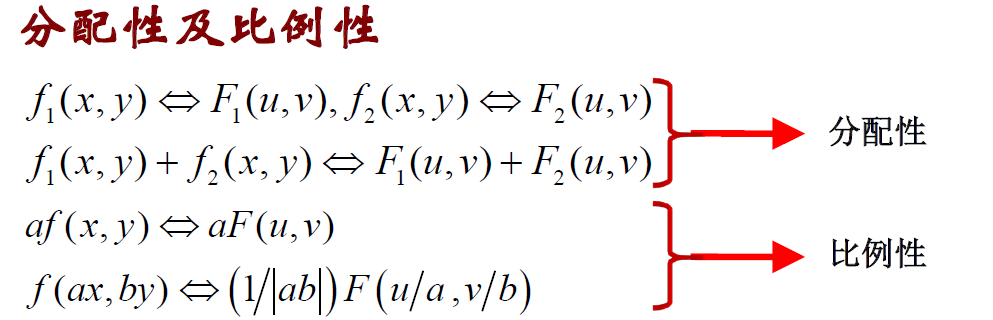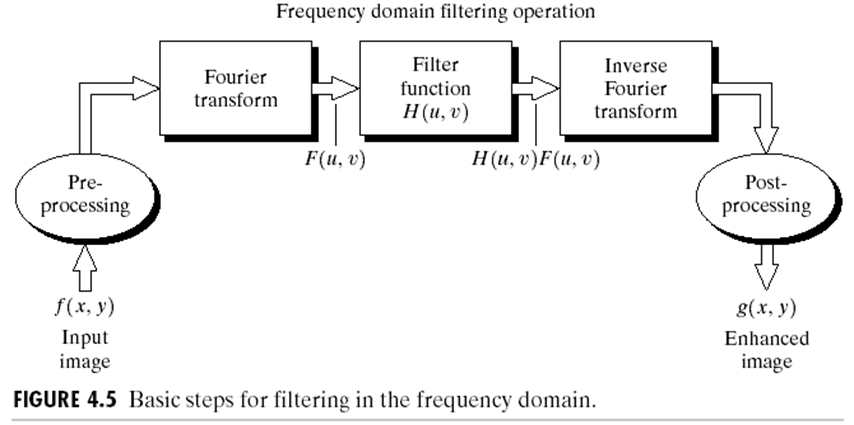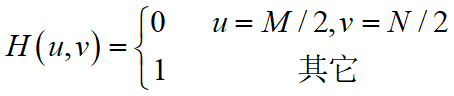# 一、图像傅里叶变换的物理意义

• 图像的频率是表征图像中灰度变化剧烈程度的指标，是灰度在平面空间上的梯度。傅里叶变换是将图像从空间域转换到频率域，换句话说，是将图像的灰度分布函数变换为图像的频率分布函数。
• 傅里叶频谱图上我们看到的明暗不一的亮点，其意义是指图像上某一点与邻域点差异的强弱，即梯度的大小，也即该点的频率的大小。
• 对频谱移频到原点以后，可以看出图像的频率分布是以原点为圆心，对称分布的。不仅可以清晰地看出图像频率分布，还可以分离出有周期性规律的干扰信号。

## 1、幅度值vs相位

$\quad$相位包含图像位置信息，幅度只是亮度，故相位包含的信息更多。

• 幅度谱告诉我们图像中某种频率的成份有多少。
• 相位谱告诉我们频率成份位于图像的什么位置。

## 2、一维连续傅里叶变换

$\quad$$f(x)$进行傅里叶变化得到$F(u)$,
$F(u)=\int_{-\infty}^{\infty}f(x)e^{-j2\pi xu}dx=R(u)+jI(u)$
$幅值：|F(u)|=\sqrt{R^2(u)+I^2(u)}$
$相位：\theta(u)=\arctan(\frac{I(u)}{R(u)})$
$F(u)=|F(u)|e^{j\theta(u)}$

## 3、二维连续傅里叶变换

$F(u,v)=\int_{-\infty}^{\infty}\int_{-\infty}^\infty f(x,y)e^{-j2\pi (ux+vy)}dxdy$

## 4、傅里叶变换的性质

• 平移特性：空域坐标移动，频域只发生相位变化，幅值不变。
• 旋转特性：空域图像旋转角度对应于频域DFT函数旋转相同角度。
•• 周期性
• 共轭对称性
• 卷积特性：时域相乘、频域相卷；反之亦然

# 二、频域滤波

$\quad$频域图像增强是指通过对图像进行傅立叶变换，将图像从空间域变换到频域，并对图像的频率成分进行相应处理，从而实现图像增强的功能。

$G(u,v)=F(u.v)H(u,v)$
$\quad$最后将$G(u,v)$进行IDFT变换即可得到频域滤波后的图像。$\quad$接下来描述三种滤波器类型。

## 1、陷波滤波器• 设置F(0,0)=0(结果图像的平均值为零)，而保留其它傅里叶变换的频率成分不变。
• 除了原点处有凹陷外，其它均是常量函数。
• 由于图像平均值为0而产生整体平均灰度级的降低。
• 用于识别由特定的、局部化频域成分引起的空间图像效果。

## 2、低通（平滑）滤波器

$\quad$被低通滤波的图像比原始图像少尖锐的细节部分而突出平滑过渡部分,对比空间域滤波的平滑处理，如均值滤波器。

## 3、高通（锐化）滤波器

$\quad$被高通滤波的图像比原始图像少灰度级的平滑过渡而突出边缘等细节部分。对比空间域的梯度算子、拉普拉斯算子。

## 4、性质

• 频域滤波器越窄，滤除的低频部分越多，图像越模糊。意味着在空域中滤波器越宽，模板就越大(阶数越高)。©️2019 CSDN 皮肤主题: 终极编程指南 设计师: CSDN官方博客